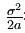# R语言对Hull White短期利率模型仿真

Wt是风险中性框架下的维纳过程，模拟随机市场风险因素。σ是标准差参数，影响利率的波动，波动幅度有着瞬时随机流动的特征。

b:长期平均水平。在长期水平下产生一系列r的轨道值。

a:回归速度。代表b的轨道值实时重组的速度。

σ：代表瞬时波动，测量每个时点随机因素进入系统的振幅​:长期方差。计算在长期所有r值围绕平均值重组的轨道值。

aσ数值相反波动：增加σ会增加随机数进入系统的数量，

a增加会使方差稳定，围绕长期平均值b以方差值波动。这在看长期方差时十分明显。 当方差值不变时，若σ增加,a减少。此模型是一个奥恩斯坦 – 乌伦贝克随机过程。

＃清理工作区

``rm(list=ls())``

＃模拟的频率

``````freq <- "monthly"

delta_t <- 1/12``````

＃数据

``````params <- list(tradeDate=as.Date('2002-2-15'),

settleDate=as.Date('2002-2-19'),

payFixed=TRUE,

dt=delta_t,

strike=.06,

method="HWAnalytic",

interpWhat="zero",

interpHow= "spline")``````

＃构建利率期限结构的市场数据

＃存款和掉期

``````tsQuotes <- list(d1w =0.0382,

s2y = 0.037125,

s3y =0.0398,``````

＃具有相应期限和期限的掉期波动率矩阵

``````swaptionMaturities <- c(1:5)

swapTenors <- c(1:5)
``````

＃为掉期定价

``````pric <- Swaption(params, swaptionMaturities, swapTenors,
``````

＃构建利率的即期期限结构

＃根据输入的市场数据

``````times <- seq(from = delta_t, to = 5, by = delta_t)

maturities <- curves\$times
``````

# 用R语言用Nelson Siegel和线性插值模型对债券价格和收益率建模

############## Hull-White短期利率模拟

＃模拟次数，频率

``````horizon <- 5
sims <- 10000``````

＃校准Hull-White参数

``````a <- pricing\$a

sigma <- pricing\$sigma``````

＃使用模拟高斯冲击

``simshos(n = nb.sims, horizon = horizon )``

＃使用模拟因子x

＃我使用远期汇率。由于每月的频率较低，

＃我认为它们是瞬时远期汇率

``````fwdrates <- ts(replicate(nb.sims, curves\$forwards),

start = start(x),

deltat = deltat(x))``````

＃ α

``  alpha <- fwdrates + param.alpha``

＃短期利率

``r <- x + alpha``

＃随机贴现因子（当前的数值积分是非常基本的）

＃由随机贴现因子得出的蒙特卡洛价格和零利率

`` montecarlozerorates <- -log(montecarloprices)/maturities ``

＃市场和蒙特卡洛价格之间的差异的置信区间

``conf.int <- t(apply((Dt - marketprices)[-1, ], 1, function(x) t.test(x)\$conf.int)) ``
``par(mfrow = c(2, 2)) ``

＃短期利率分位数

＃蒙特卡洛vs市场零利率

``````plot(maturities, montecarlozerorates, type='l', col = 'blue', lwd = 3,

points(maturities, marketzerorates, col = 'red')``````

＃蒙特卡洛vs市场零息价格

``````plot(maturities, montecarloprices, type ='l', col = 'blue', lwd = 3,

points(maturities, marketprices, col = 'red')``````

＃价格差的置信区间

``````matplot(maturities[-1], conf.int, type = 'l',

``````

Kaizong Ye拓端研究室（TRL）的研究员。

​非常感谢您阅读本文，如需帮助请联系我们！QQ在线咨询

15121130882

0571-63341498

## 关注有关新文章的微信公众号

This will close in 0 seconds